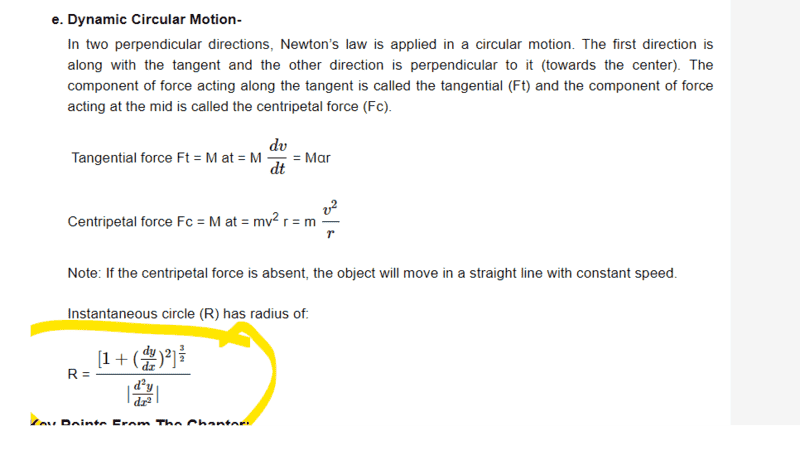# Dynamic circular motion

• Nway
In summary, the author tries to show that the instantaneous circle is given by the formula when there is no centripetal force, but is unsuccessful.

#### Nway

Homework Statement
I am trying to understand circular motion from IIT JEE curriculum.
Relevant Equations
Below.
Can someone show that the instantaneous circle is indeed given by when the centripetal force is removed?This can be found at https://www.vedantu.com/iit-jee/circular-motion

That page seems quite confused. In general, dynamic circular motion requires an object to be constrained to move in a circle of given radius.

The orbit of a satellite in general is an ellipse. If a satellite is in a circular orbit and you give it a tangential boost, it will transition to an elliptical orbit, not to a smaller circular orbit.

Also, the formula you circled relates the decomposition of forces for an arbitrary (not necessarily circular) trajectory into instantaneous tangential and normal (centripetal components).

•Nway
Nway said:
Can someone show that the instantaneous circle is indeed given by when the centripetal force is removed?
View attachment 320998This can be found at https://www.vedantu.com/iit-jee/circular-motion
First let's correct this, which is obviously wrong:
Fc = M at = mv2 r = ##m \frac{v^2}r##
Presumably they meant ##F_c = M at = m\omega^2 r = m \frac{v^2}r##.

I interpret your question as "show that the instantaneous circle is indeed given by the formula circled when there is no centripetal force".
Easy. If there is no force normal to the velocity then the motion is a straight line. ##\frac{d^2y}{dx^2}=0##, so R is infinite.

As for the rest of that web page, it's pretty shoddy.
E.g.:
Some examples of non-uniform circular motion:
1. A bouncing ball.
Eh?
Changes that take place in the speed have implications for radial acceleration. There may be two possibilities that are as follows:
This simply means that the centripetal acceleration is nonstop.
What does that mean?

### What is a Non-Uniform Circular Motion? ​

In another possibility, the radial (centripetal) force is constant…. This simply means that unlike in the case of uniform motion, the radius of the circular path is variable.
Then it's not circular.
• Both centrifugal and centripetal forces are equal in magnitude and opposite in directions.
• These both centripetal and centrifugal forces cannot be termed as action and reaction since action and reaction never act on the same body.
That's really confusing. It makes it sound as though both operate always.
In any frame, centripetal force is that component of the net force which acts normal to the velocity.
In an inertial frame, there is no centrifugal force.
In a frame rotating with the body (making it appear to move in a straight line) we find that Newton’s laws appear to be violated. We observe a lateral force being exerted on it (gravity, a string..) yet the motion is straight. To explain this we add a fictitious radially outward force, centrifugal force.

I don't want to overcomplicate things, but we could choose a frame that rotates, though not exactly with the object. We would then have a centrifugal force as one of the contributors to the net force, of which the centripetal force would be the component normal to the velocity!
So there are two key differences between centrifugal and centripetal. Centripetal force is not an applied force; it is a component of the resultant of the applied forces (real or fictitious).
Centrifugal force is an applied force, but it is "fictitious".
PeroK said:
the formula you circled relates the decomposition of forces for an arbitrary (not necessarily circular) trajectory into instantaneous tangential and normal (centripetal components).
We must be looking at different circled formulae.

•Nway
•Nway
PeroK said:
I recognised that formula as the reciprocal of the curvature, ##\kappa##, of any smooth curve, as defined here:

https://tutorial.math.lamar.edu/classes/calciii/curvature.aspx
Sure, but how does it relate to decomposition of forces? (Beyond being the formula for radius of curvature, as stated.)

•Nway
haruspex said:
Sure, but how does it relate to decomposition of forces?
The issue is that page is no longer talking about circular motion but about general motion with tangential and centripetal components.

The material is explicitly under the heading "dynamic circular motion", which is wrong, IMO.

•Nway
PeroK said:
The issue is that page is no longer talking about circular motion but about general motion with tangential and centripetal components.

The material is explicitly under the heading "dynamic circular motion", which is wrong, IMO.
Yes, it's a mess.

•Nway, SammyS and nasu